# Applications of Crystal Field Theory Ionic Radii expe

• Slides: 8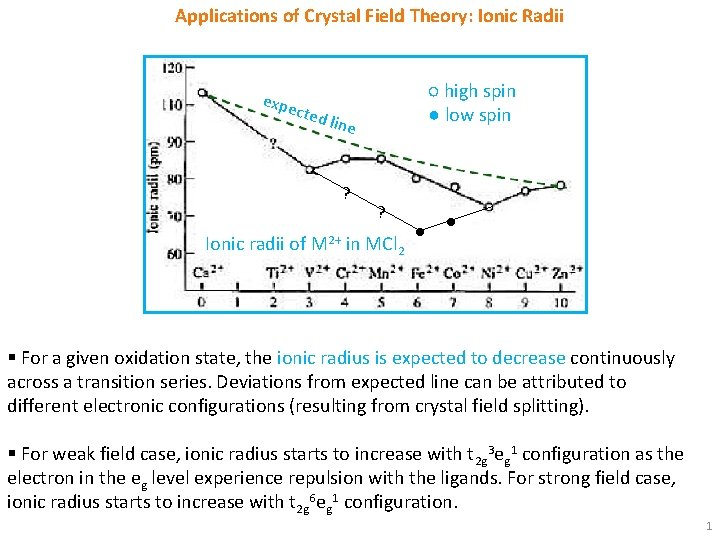Applications of Crystal Field Theory: Ionic Radii expe cted ○ high spin ● low spin line ? ? Ionic radii of M 2+ in MCl 2 ● ● § For a given oxidation state, the ionic radius is expected to decrease continuously across a transition series. Deviations from expected line can be attributed to different electronic configurations (resulting from crystal field splitting). § For weak field case, ionic radius starts to increase with t 2 g 3 eg 1 configuration as the electron in the eg level experience repulsion with the ligands. For strong field case, ionic radius starts to increase with t 2 g 6 eg 1 configuration. 1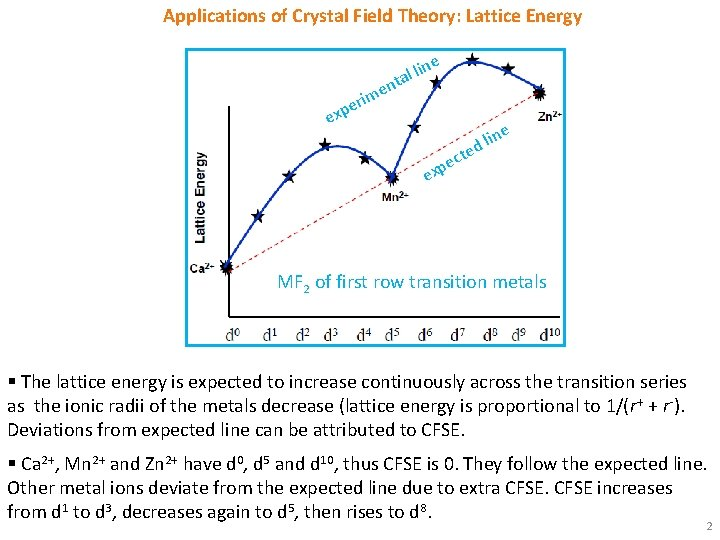Applications of Crystal Field Theory: Lattice Energy l lin a t n e e ex im r e p ne i l d e ct e p x e MF 2 of first row transition metals § The lattice energy is expected to increase continuously across the transition series as the ionic radii of the metals decrease (lattice energy is proportional to 1/(r+ + r-). Deviations from expected line can be attributed to CFSE. § Ca 2+, Mn 2+ and Zn 2+ have d 0, d 5 and d 10, thus CFSE is 0. They follow the expected line. Other metal ions deviate from the expected line due to extra CFSE increases from d 1 to d 3, decreases again to d 5, then rises to d 8. 2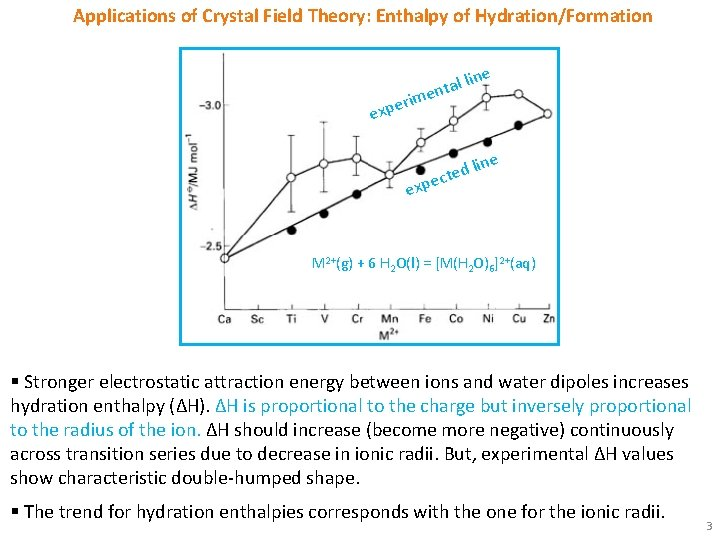Applications of Crystal Field Theory: Enthalpy of Hydration/Formation tal li n e rim ne expe ne d li e t c xpe e M 2+(g) + 6 H 2 O(l) = [M(H 2 O)6]2+(aq) § Stronger electrostatic attraction energy between ions and water dipoles increases hydration enthalpy (ΔH). ΔH is proportional to the charge but inversely proportional to the radius of the ion. ΔH should increase (become more negative) continuously across transition series due to decrease in ionic radii. But, experimental ΔH values show characteristic double-humped shape. § The trend for hydration enthalpies corresponds with the one for the ionic radii. 3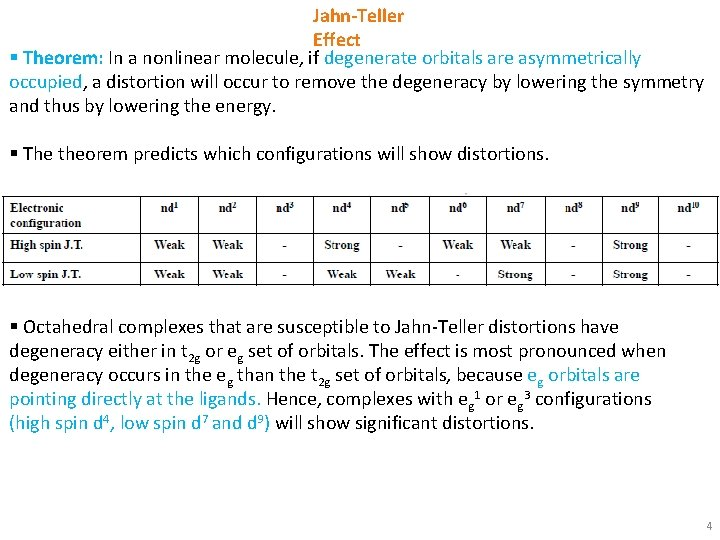Jahn-Teller Effect § Theorem: In a nonlinear molecule, if degenerate orbitals are asymmetrically occupied, a distortion will occur to remove the degeneracy by lowering the symmetry and thus by lowering the energy. § The theorem predicts which configurations will show distortions. § Octahedral complexes that are susceptible to Jahn-Teller distortions have degeneracy either in t 2 g or eg set of orbitals. The effect is most pronounced when degeneracy occurs in the eg than the t 2 g set of orbitals, because eg orbitals are pointing directly at the ligands. Hence, complexes with eg 1 or eg 3 configurations (high spin d 4, low spin d 7 and d 9) will show significant distortions. 4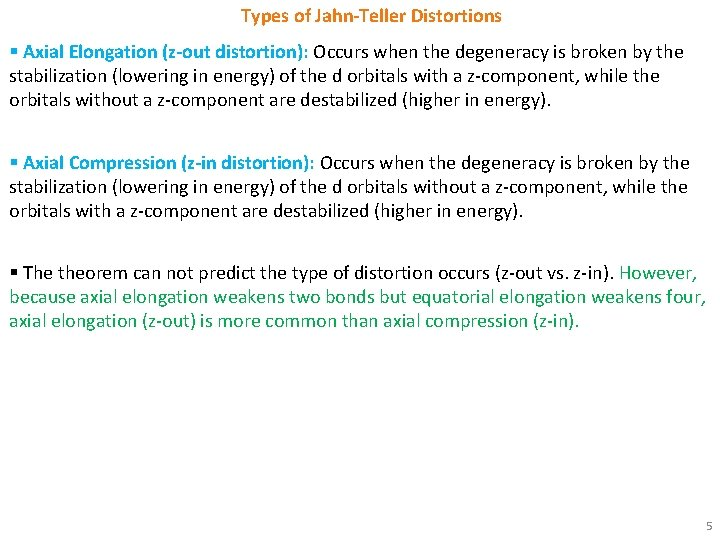Types of Jahn-Teller Distortions § Axial Elongation (z-out distortion): Occurs when the degeneracy is broken by the stabilization (lowering in energy) of the d orbitals with a z-component, while the orbitals without a z-component are destabilized (higher in energy). § Axial Compression (z-in distortion): Occurs when the degeneracy is broken by the stabilization (lowering in energy) of the d orbitals without a z-component, while the orbitals with a z-component are destabilized (higher in energy). § The theorem can not predict the type of distortion occurs (z-out vs. z-in). However, because axial elongation weakens two bonds but equatorial elongation weakens four, axial elongation (z-out) is more common than axial compression (z-in). 5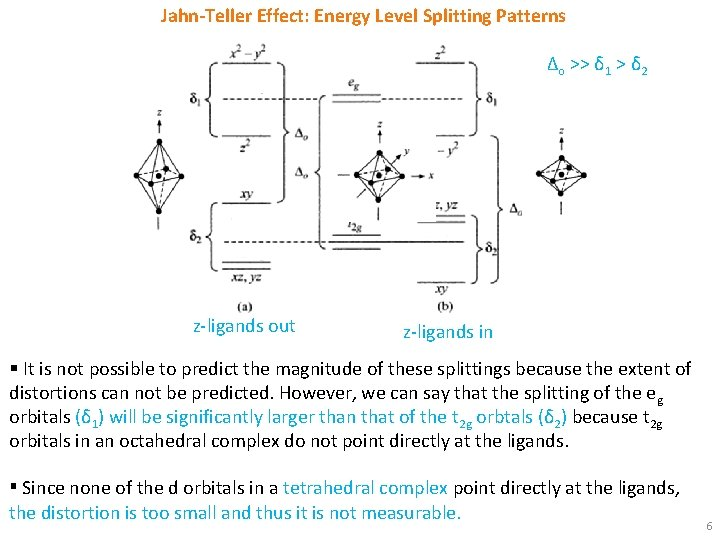Jahn-Teller Effect: Energy Level Splitting Patterns Δo >> δ 1 > δ 2 z-ligands out z-ligands in § It is not possible to predict the magnitude of these splittings because the extent of distortions can not be predicted. However, we can say that the splitting of the e g orbitals (δ 1) will be significantly larger than that of the t 2 g orbtals (δ 2) because t 2 g orbitals in an octahedral complex do not point directly at the ligands. ▪ Since none of the d orbitals in a tetrahedral complex point directly at the ligands, the distortion is too small and thus it is not measurable. 6Jahn-Teller Effect: Explanation § If one or three electrons occupy the eg orbitals (as in high-spin d 4, low-spin d 7 and d 9 complexes) a tetragonal distortion may be energetically advantageous. § For example, in a high spin d 4 ion (t 2 g 3 eg 1), if the last electron occupies dz 2 orbital, most of the electron density will be concentrated between the cation and the two ligands on the z axis. Thus, there will be greater electrostatic repulsion associated with these ligands than with the other four ligands and the complex suffers elongation. Conversely, if the last electron occupies dx 2 -y 2 orbital, elongation will occur along x and y axes. Similar situations will arise for a low spin d 7 ion (t 2 g 6 eg 1). § Another example: for the d 9 configuration, two orbitals in the eg set are occupied by one and two electrons, respectively. If two electrons occupies d z 2 orbital and the third electron occupies dx 2 -y 2 orbital, most of the electron density will be concentrated between the cation and the two ligands on the z axis. Thus, there will be greater electrostatic repulsion associated with these ligands than with the other four ligands and the complex suffers elongation. Conversely, if two electrons occupies dx 2 -y 2 orbital and the third electron occupies dz 2 orbital, elongation will occur along x and y axes. 7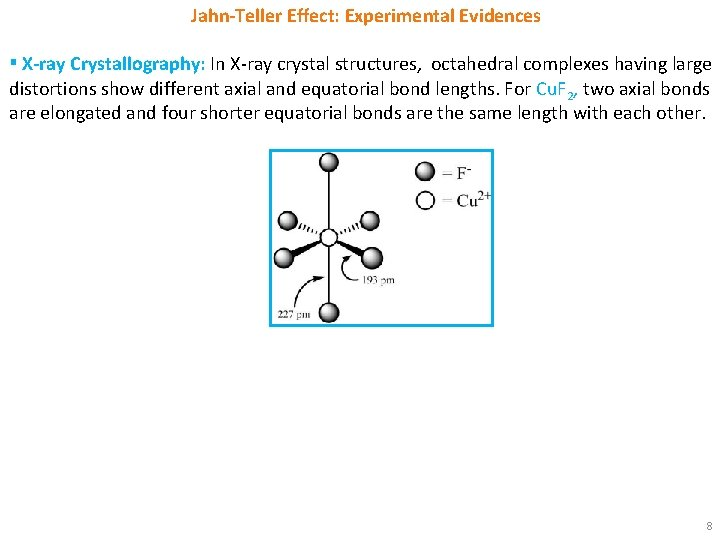Jahn-Teller Effect: Experimental Evidences ▪ X-ray Crystallography: In X-ray crystal structures, octahedral complexes having large distortions show different axial and equatorial bond lengths. For Cu. F 2, two axial bonds are elongated and four shorter equatorial bonds are the same length with each other. 8